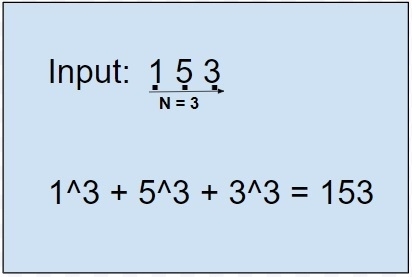# C Program to check Plus Perfect Number

Given a number x with n number of digits, our task is to check whether the given number’s Plus Perfect number or not. In order to check that the number is Plus Perfect Number we find the nth power of every digit d (d^n) and then sum all the digits, if the sum is equal to n then the number is Plus Perfect Number. Plus Perfect number is similar like finding an Armstrong of any number.

Like In the given example below −## Example

Input: 163
Output: Number is not a perfect_number
Explanation: 1^3 + 6^3 + 3^3 is not equal to 163
Input: 371
Output: Number is a perfect_number
Explanation: 3^3 + 7^3 +1^3 is equal to 371

The approach used below is as follows

• First step is to count the number of digits in the input given.
• Second step is to power a digit the same number of times as the number of digits of the input.
• Third step is adding all the numbers and check if it is equal or not.

## Algorithm

Start
In function int power(int a, int b)
Step 1-> Declare and initialize power as 1
Step 2-> Loop While b>0
Set power = power * a
Decrement b by 1
Step 3-> return power
End function power
In function int count(int n)
Step 1-> Declare and Initialize i as 0
Step 2-> Loop While n!=0
Increment i by 1
Set n = n/10
End Loop
Step 3-> Return i
In function int perfect_number(int n)
Step 1-> Declare and initialize x as count(n)
Step 2-> Declare and initialize rem as 0 and m as 0
Step 3-> Loop While(n)
Set rem as n %10
Set m as m + power(rem, x)
Set n as n/ 10
End Loop
Step 4-> Return m
End Function perfect_number
In Function int main(int argc, char const *argv[])
Step 1-> Initialize n as 1634
Step 2-> If n == perfect_number(n) then,
Print "Number is a perfect_number "
Step 3-> else
Print "Number is not a perfect_number "
End if
End main
Stop

## Example

Live Demo

#include <stdio.h>
int power(int a, int b) {
int power =1;
while(b>0) {
power *= a;
b--;
}
return power;
}
int count(int n) {
int i=0;
while(n!=0) {
i++;
n = n/10;
}
return i;
}
int perfect_number(int n) {
int x = count(n);
int rem = 0, m=0;
while(n) {
rem = n %10;
m += power(rem, x);
n /= 10;
}
return m;
}
int main(int argc, char const *argv[]) {
int n = 1634;
if(n == perfect_number(n)) {
printf("Number is a perfect_number");
}
else
printf("Number is not a perfect_number");
return 0;
}

## Output

If run the above code it will generate the following output −

Number is a perfect_number